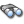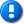alvinalexander.com | career | drupal | java | mac | mysql | perl | scala | uml | unix

# Commons Math example source code file (MixedListUnivariateImplTest.java)

This example Commons Math source code file (MixedListUnivariateImplTest.java) is included in the DevDaily.com "Java Source Code Warehouse" project. The intent of this project is to help you "Learn Java by Example" TM.

## Java - Commons Math tags/keywords

arraylist, arraylist, bar, descriptivestatistics, foo, io, listunivariateimpl, listunivariateimpl, mixedlistunivariateimpltest, nan, nan, numbertransformer, serializable, transformermap, transformermap, util

## The Commons Math MixedListUnivariateImplTest.java source code

```/*
* Licensed to the Apache Software Foundation (ASF) under one or more
* contributor license agreements.  See the NOTICE file distributed with
* The ASF licenses this file to You under the Apache License, Version 2.0
* (the "License"); you may not use this file except in compliance with
* the License.  You may obtain a copy of the License at
*
*
* Unless required by applicable law or agreed to in writing, software
* WITHOUT WARRANTIES OR CONDITIONS OF ANY KIND, either express or implied.
* See the License for the specific language governing permissions and
*/
package org.apache.commons.math.stat.descriptive;

import java.io.Serializable;
import java.util.ArrayList;
import java.util.List;

import junit.framework.TestCase;

import org.apache.commons.math.util.NumberTransformer;
import org.apache.commons.math.util.TransformerMap;

/**
* Test cases for the {@link ListUnivariateImpl} class.
*
* @version \$Revision: 902201 \$ \$Date: 2010-01-22 13:18:16 -0500 (Fri, 22 Jan 2010) \$
*/

public final class MixedListUnivariateImplTest extends TestCase {
private double one = 1;
private float two = 2;
private int three = 3;

private double mean = 2;
private double sumSq = 18;
private double sum = 8;
private double var = 0.666666666666666666667;
private double std = Math.sqrt(var);
private double n = 4;
private double min = 1;
private double max = 3;
private double tolerance = 10E-15;

private TransformerMap transformers = new TransformerMap();

public MixedListUnivariateImplTest(String name) {
super(name);
transformers = new TransformerMap();

transformers.putTransformer(Foo.class, new FooTransformer());

transformers.putTransformer(Bar.class, new BarTransformer());

}

/** test stats */
public void testStats() {
List<Object> externalList = new ArrayList();

DescriptiveStatistics u = new ListUnivariateImpl(externalList,transformers);

assertEquals("total count", 0, u.getN(), tolerance);
assertEquals("N", n, u.getN(), tolerance);
assertEquals("sum", sum, u.getSum(), tolerance);
assertEquals("sumsq", sumSq, u.getSumsq(), tolerance);
assertEquals("var", var, u.getVariance(), tolerance);
assertEquals("std", std, u.getStandardDeviation(), tolerance);
assertEquals("mean", mean, u.getMean(), tolerance);
assertEquals("min", min, u.getMin(), tolerance);
assertEquals("max", max, u.getMax(), tolerance);
u.clear();
assertEquals("total count", 0, u.getN(), tolerance);
}

public void testN0andN1Conditions() throws Exception {
DescriptiveStatistics u = new ListUnivariateImpl(new ArrayList<Object>(),transformers);

assertTrue(
"Mean of n = 0 set should be NaN",
Double.isNaN(u.getMean()));
assertTrue(
"Standard Deviation of n = 0 set should be NaN",
Double.isNaN(u.getStandardDeviation()));
assertTrue(
"Variance of n = 0 set should be NaN",
Double.isNaN(u.getVariance()));

assertTrue(
"Mean of n = 1 set should be value of single item n1, instead it is " + u.getMean() ,
u.getMean() == one);

assertTrue(
"StdDev of n = 1 set should be zero, instead it is: "
+ u.getStandardDeviation(),
u.getStandardDeviation() == 0);
assertTrue(
"Variance of n = 1 set should be zero",
u.getVariance() == 0);
}

public void testSkewAndKurtosis() {
ListUnivariateImpl u =
new ListUnivariateImpl(new ArrayList<Object>(), transformers);

assertEquals("mean", 12.40455, u.getMean(), 0.0001);
assertEquals("variance", 10.00236, u.getVariance(), 0.0001);
assertEquals("skewness", 1.437424, u.getSkewness(), 0.0001);
assertEquals("kurtosis", 2.37719, u.getKurtosis(), 0.0001);
}

public void testProductAndGeometricMean() throws Exception {
ListUnivariateImpl u = new ListUnivariateImpl(new ArrayList<Object>(),transformers);
u.setWindowSize(10);

assertEquals(
"Geometric mean not expected",
2.213364,
u.getGeometricMean(),
0.00001);

// Now test rolling - StorelessDescriptiveStatistics should discount the contribution
for (int i = 0; i < 10; i++) {
}
// Values should be (2,3,4,5,6,7,8,9,10,11)
assertEquals(
"Geometric mean not expected",
5.755931,
u.getGeometricMean(),
0.00001);

}

public static final class Foo {
public String heresFoo() {
return "14.9";
}
}

public static final class FooTransformer implements NumberTransformer, Serializable {
private static final long serialVersionUID = -4252248129291326127L;
public double transform(Object o) {
return Double.parseDouble(((Foo) o).heresFoo());
}
}

public static final class Bar {
public String heresBar() {
return "12.0";
}
}

public static final class BarTransformer implements NumberTransformer, Serializable {
private static final long serialVersionUID = -1768345377764262043L;
public double transform(Object o) {
return Double.parseDouble(((Bar) o).heresBar());
}
}

}

Other Commons Math examples (source code examples)
Here is a short list of links related to this Commons Math MixedListUnivariateImplTest.java source code file:The search pageOther Commons Math source code examples at this package levelClick here to learn more about this project

```
 ... this post is sponsored by my books ...#1 New Release!FP Best Seller